NEET  >  Test: Chemical Equilibrium: Law of Mass Action

# Test: Chemical Equilibrium: Law of Mass Action

Test Description

## 21 Questions MCQ Test Chemistry Class 11 | Test: Chemical Equilibrium: Law of Mass Action

Test: Chemical Equilibrium: Law of Mass Action for NEET 2022 is part of Chemistry Class 11 preparation. The Test: Chemical Equilibrium: Law of Mass Action questions and answers have been prepared according to the NEET exam syllabus.The Test: Chemical Equilibrium: Law of Mass Action MCQs are made for NEET 2022 Exam. Find important definitions, questions, notes, meanings, examples, exercises, MCQs and online tests for Test: Chemical Equilibrium: Law of Mass Action below.
Solutions of Test: Chemical Equilibrium: Law of Mass Action questions in English are available as part of our Chemistry Class 11 for NEET & Test: Chemical Equilibrium: Law of Mass Action solutions in Hindi for Chemistry Class 11 course. Download more important topics, notes, lectures and mock test series for NEET Exam by signing up for free. Attempt Test: Chemical Equilibrium: Law of Mass Action | 21 questions in 30 minutes | Mock test for NEET preparation | Free important questions MCQ to study Chemistry Class 11 for NEET Exam | Download free PDF with solutions
 1 Crore+ students have signed up on EduRev. Have you?
Test: Chemical Equilibrium: Law of Mass Action - Question 1

### Direction (Q. Nos. 1-12) This section contains 12 multiple choice questions. Each question has four choices (a), (b), (c) and (d), out of which ONLY ONE option is correct.   Q.Conversion factor for converting partial pressures (in Kp) to active masses (in Kc) is

Detailed Solution for Test: Chemical Equilibrium: Law of Mass Action - Question 1

Kp = kc(RT)∆ng
So to convert kp to kc we have, kc = kp(1/RT)∆ng.
So, the converting factor is 1/RT

Test: Chemical Equilibrium: Law of Mass Action - Question 2

### For which of the following reactions does the equilibrium constant depends on the unit of concentration?

Detailed Solution for Test: Chemical Equilibrium: Law of Mass Action - Question 2

For the reaction COCl2 (g) ⇌ CO(g) + Cl2 (g), the equilibrium constant depends on the units of concentration.
For this reaction, the number of moles of reactants is not equal to the number of moles of products. So in the equilibrium constant expression, the units of concentration do not cancel out.
K = [CO] [Cl2] ÷ [COCl2]
K = mol/L × mol/L ÷ mol/L = mol/L

Test: Chemical Equilibrium: Law of Mass Action - Question 3

### The concentration of the oxides of nitrogen are monitored in air-pollution reports. At 25°C, the equilibrium constant for the reaction,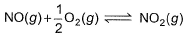is 1.3 x 106 and that for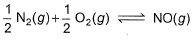is 6.5 x 10-16 (when each species is expressed in terms of partial pressure). For the reaction,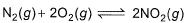equilibrium constant is

Detailed Solution for Test: Chemical Equilibrium: Law of Mass Action - Question 3

Given equations are
NO (g) + ½ O2 (g) ⇌ NO2 (g) ----------(i) k1 = 1.3×106
And ½ N2 (g) + ½ O2 (g)     ⇌     NO(g) ----------(ii) k2 = 6.5×10-16
To get the reaction, N2(g) + 2O2(g) ⇌ 2NO2(g) ----------(iii) k3
We multiply eqn (i) and eqn (ii) by 2 and adding both reaction, we get eqn (iii)
k3 = k12×k22
= (1.3×106)2×(6.5×10-16)2
= 1.69×1012×42.25×10-32
= 7.14×10-19

Test: Chemical Equilibrium: Law of Mass Action - Question 4

For the following gaseous phase equilibrium,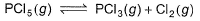Kp is found to be equal to Kx (Kx is equilibrium constant when concentration are taken in terms of mole fraction. This is attained when pressure is

Detailed Solution for Test: Chemical Equilibrium: Law of Mass Action - Question 4

The correct answer is Option A.
Kp = Equilibrium constant in terms of partial pressure
Kc = Equilibrium constant in terms of concentration
Kx = Equilibrium constant in terms of mole fraction
Kp = KcRTΔn ---(1)
Kp = K * (Pt)Δn ---(2)
a)   1 atm
Given PCl5 (g) ---> PCl3 (g) + Cl2 (g)
Δn = 2 – 1
Given Kp = Kx
From (2)
Kp = Kx when PT = 1

Test: Chemical Equilibrium: Law of Mass Action - Question 5

For the reaction in equilibrium, A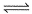B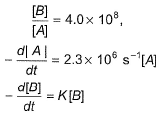Thus, K is

Detailed Solution for Test: Chemical Equilibrium: Law of Mass Action - Question 5

From the  reaction
-d[A]/dt = d[B] /dt
⇒2.3 × 106 [A] = k [B]
as given in question [B] /[A] = 4 × 108
so [A] / [B] = 1/ 4 ×108
⇒ 2.3 × 106 . [A] /[B] = k
⇒  2.3 × 106 / 4 × 108 = k
Or k = 5.8 × 10-3 /sec¹

Test: Chemical Equilibrium: Law of Mass Action - Question 6

For the following equilibrium, N2O4 (g)2NO2(g)

Kp = KC. This is attained when

Detailed Solution for Test: Chemical Equilibrium: Law of Mass Action - Question 6

The correct answer is option B
KP​=KC​(RT)Δn
Δn=1
KP​=KC​(RT)
RT=1
T=1/R=1/0.0821=12.18

Test: Chemical Equilibrium: Law of Mass Action - Question 7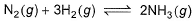is a gaseous phase equilibrium reaction taking place at 400 K in a 5 L flask. For this

Detailed Solution for Test: Chemical Equilibrium: Law of Mass Action - Question 7

Test: Chemical Equilibrium: Law of Mass Action - Question 8

A sample of pure PCI5(g)was introduced into an evacuated vessel at 473 K. After equilibrium was attained, concentration of PCI5 was found to be 0.05 mol L-1.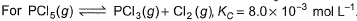Thus, [PCI3] and [Cl2](in mol L-1)at equilibrium are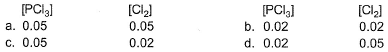Detailed Solution for Test: Chemical Equilibrium: Law of Mass Action - Question 8

The correct answer is Option B
Let x M be the equilibrium concentration of PCl3​.
The equilibrium reaction is shown below.
PCl5​(g) ⇌ PCl3​(g) + Cl2​(g)
The equilibrium concentrations of PCl5​, PCl3​ and Cl2​ are 0.5×10−2M, x and x respectively.
The expression of the equilibrium constant is
Kc​= ( [PCl3​][Cl2​] ​) / [PCl3​].
Substitute values in the above expression.
8.3 × 10−3  = x2 / (0.5 × 10−1​)
x2 = 4.15m × 10−4
x ≃ 0.02M.

Test: Chemical Equilibrium: Law of Mass Action - Question 9

Ag+(aq)+NH3(aq)[Ag(NH3)(aq)]+ ; K, = 3.5x 10-3
[Ag(NH3)]+ (aq)+NH3(aq)[Ag(NH3)2]2+(aq); K2 = 1.7x 10-3
Formation constant of [Ag(NH3)2]+(aq) is

Detailed Solution for Test: Chemical Equilibrium: Law of Mass Action - Question 9

The correct answer is Option C.
To get the formation constant add both reactions-
So the resultant K = K1 × K2
= 3.5×1.7×10−3
= 5.95×10−6

Test: Chemical Equilibrium: Law of Mass Action - Question 10

For the reversible reaction,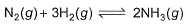at 500° C, the value of Kp is 1.44x 10-5, when partial pressure is measured in atmosphere. The corresponding value of Kc with concentration in mol L-1 is

[IITJEE 2000]

Detailed Solution for Test: Chemical Equilibrium: Law of Mass Action - Question 10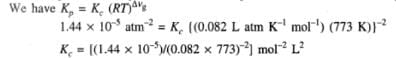Test: Chemical Equilibrium: Law of Mass Action - Question 11

For the reaction,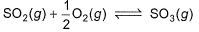if Kp = Kc (RT)X, when the symbols have usual meaning, the value of x is (assuming ideality)

[jee Main 2014]

Detailed Solution for Test: Chemical Equilibrium: Law of Mass Action - Question 11

The correct answer is Option B.
SO2(g) + 1/2O2(g) ⇌ SO3(g)
KP = KC(RT)Δn

Δn= no. of gaseous moles of product minus no. of gaseous moles of reactant
Δn = 1−1−1/2
∴Δn = −1/2

Test: Chemical Equilibrium: Law of Mass Action - Question 12

Given that for the equilibrium constants of two reactions,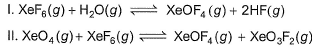areK1 and K2. Equilibrium constant k3 of the following reaction in terms of k1, and K2.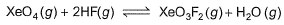Detailed Solution for Test: Chemical Equilibrium: Law of Mass Action - Question 12

The correct answer is Option C
XeF6(g) + H2O(g) ⇌ XeOF4(g)+2HF(g)

K1 = ([XeOF4][HF]2 ) / ( [XeF6][H2O] ) ...(i)

XeO4(g) + XeF6(g) ⇌ XeOF4(g) + XeO3F2(g)

K2 = ([XeOF4][XeO3F2] ) / ( [XeO4][XeF6] )  ...(ii)

For the reaction,

XeO4(g) + 2HF(g) ⇌ XeO3F2(g) + H2O(g)

K=[XeO3F2][H2O]) / ([XeO4][HF]2 )  ...(iii)

∴ From Eqs. (i), (ii) and (iii)

K= K2 / K1

*Multiple options can be correct
Test: Chemical Equilibrium: Law of Mass Action - Question 13

Direction (Q. Nos. 13-14) This section contains 4 multiple choice questions. Each question has four choices (a), (b), (c) and (d), out of which ONE or  MORE THANT ONE  is correct.

For the following type of reversible reaction,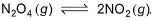*Multiple options can be correct
Test: Chemical Equilibrium: Law of Mass Action - Question 14

Select the correct statement (s) about the following equilibrium.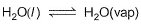Detailed Solution for Test: Chemical Equilibrium: Law of Mass Action - Question 14

The correct answers are Options A, B and C.
During constant vapor pressure the no of molecules of H2O leaving the surface = the no of H2O molecules coming to the surface from the atmosphere. This process is dynamic as there is continuous movement of molecules.

Test: Chemical Equilibrium: Law of Mass Action - Question 15

Direction (Q. Nos. 15-18) This section contains  a paragraph, wach describing theory, experiments, data etc. three Questions related to paragraph have been given.Each question have only one correct answer among the four given options (a),(b),(c),(d)

Passage I

At 573 K, PCI5 dissociates as,
PCI5 (g) ⇔ PCI3(g)+ Cl2 (g), Kp= 11.5 atm
Given, [PCI3]eq, = [Cl2]eq = 0.01 mol L-1

Q
Kc of this equilibrium is

Detailed Solution for Test: Chemical Equilibrium: Law of Mass Action - Question 15

The correct answer is 0.27 mol.L-1
The given reversible gaseous reaction & ice  table:
PCl5(g)⇌PCl3(g) + Cl2(g)
I-  2mol            0 mol       0mol
C- 2α mol        2α mol     2α mol
E- 2(1-α ) mol  2α mol     2α mol
Where degree of dissociation, α=40% =0.4
Volume of the equilibrium mixture, V=2L
At equilibrium the molar concentrations of the components of the mixture are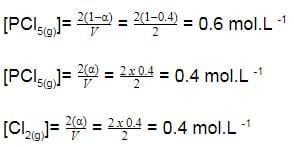Equilibrium Constant: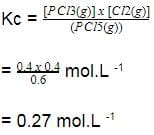Test: Chemical Equilibrium: Law of Mass Action - Question 16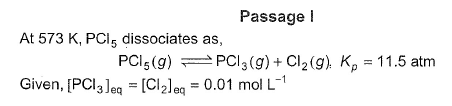Q. What is [PCI5] eq?

Detailed Solution for Test: Chemical Equilibrium: Law of Mass Action - Question 16

The correct answer is Option A.
PPCl3 = [PCl3]RT
PCl2 = [Cl2]RT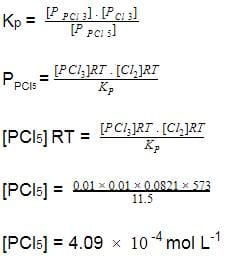Test: Chemical Equilibrium: Law of Mass Action - Question 17

Passage II

The complex ion of Fe2+ with the chelating agent dipyridyl (abbreviated dipy) has been studied kinetically in both the forward and backward directions. For the complexion reaction,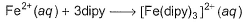the rate of the formation of the complex at 298 K is given by rate = (1. 45 x 1013 L3mol -3s-1) [Fe 2+] [dipy]3 and for the reverse reaction , the rate of disapperance of the complex is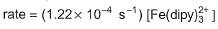Q. What is equilibrium constant for the equilibrium ?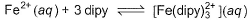Detailed Solution for Test: Chemical Equilibrium: Law of Mass Action - Question 17

The correct answer is Option C.
Fe2+ + 3 dipy -----> Fe(dipy)32+
Rf = Kf [Fe2+] [dipy]3
Rb = Kb [Fe(dipy)32+]
Keq = Kb [Fe(dipy)32+] / Kf [Fe2+] [dipy]3
Rf = Rb
Keq = Kf / Kb
= 1. 45 x 1013 / 1.24 x 10-4
= 1.885 x 1017

Test: Chemical Equilibrium: Law of Mass Action - Question 18

Passage II

The complex ion of Fe2+ with the chelating agent dipyridyl (abbreviated dipy) has been studied kinetically in both the forward and backward directions. For the complexion reaction,the rate of the formation of the complex at 298 K is given by rate = (1. 45 x 1013 L3mol -3s-1) [Fe 2+] [dipy]3 and for the reverse reaction , the rate of disapperance of the complex isQ. If half-life Period of the backward reaction is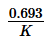(k = rate constant), then half-life period is about

*Answer can only contain numeric values
Test: Chemical Equilibrium: Law of Mass Action - Question 19

Direction (Q. Nos. 19 and 20) This section contains 2 questions. when worked out will result in an integer from 0 to 9 (both inclusive)

Q. At elevated temperature, PCl5 dissociates as,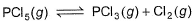At 300°C,Kp = 11.8 and
[PCI3]= [Cl2]= 0.01 moi L-1 at equilibrium.
[PCI5] = x x 10-4 mol L-1 what is the value of x?

Detailed Solution for Test: Chemical Equilibrium: Law of Mass Action - Question 19

Applying Kp = kcRT∆ng
11.8 = kc(0.0821)(273+300)(1)
Kc = 0.25
Applying Kc = [PCl3][Cl2]/[PCl5]
0.25 = 10-4 / [PCl5]
[PCl5] = 10-4/0.25
= 4×10-4
Therefore x = 4

*Answer can only contain numeric values
Test: Chemical Equilibrium: Law of Mass Action - Question 20

What is Kp for the equation,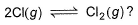When the system contains equal number of Cl (g)atom and Cl2(g) molecules at 1 bar and 300 K?

Detailed Solution for Test: Chemical Equilibrium: Law of Mass Action - Question 20

The correct answer is 2
2Cl(g) ⇌ Cl2(g)
Initially take moles of Cl on right hand side to be 1 then
2Cl           Cl2
1               -x
=1 - x.x
kP  will be equal to Kc because no. of moles are equal so
1 - x = x
2x = 1
X = ½
Kp = x/1 - x. 1 - x
= ½ ÷ (½ * ½ )
=2

Test: Chemical Equilibrium: Law of Mass Action - Question 21

Direction (Q. Nos. 21) Choice the correct combination of elements and column I and coloumn II  are given as option (a), (b), (c) and (d), out of which ONE option is correct.

The progress of the reaction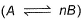with time t is shown below.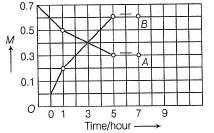Match the parameters in Column l with their respective values in Column II.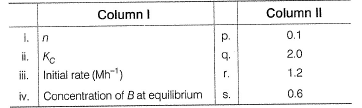Codes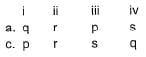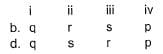Detailed Solution for Test: Chemical Equilibrium: Law of Mass Action - Question 21

The correct answer is Option A.

Loss in concentration of A in I hour =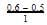= 0.1
Gain in concentration of B in I hour =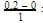0.2
(i) ∵0.1 mole of A changes to 0.2 mole of B in a given time and thus, n=2
(ii) Equilibrium constant,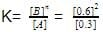= 1.2mollitre−1
(iii) Initial rate of conversion of A = changes in conc. of A during I hour =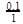= 0.1 mol litre−1hour−1
(iv) ∵ Equilibrium is attained after 5 hr, where [B]=0.6 and [A]=0.3

## Chemistry Class 11

204 videos|320 docs|229 tests
 Use Code STAYHOME200 and get INR 200 additional OFF Use Coupon Code
Information about Test: Chemical Equilibrium: Law of Mass Action Page
In this test you can find the Exam questions for Test: Chemical Equilibrium: Law of Mass Action solved & explained in the simplest way possible. Besides giving Questions and answers for Test: Chemical Equilibrium: Law of Mass Action, EduRev gives you an ample number of Online tests for practice

## Chemistry Class 11

204 videos|320 docs|229 tests

### How to Prepare for NEET

Read our guide to prepare for NEET which is created by Toppers & the best Teachers